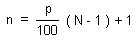HOME MATH DICTIONARY DOWNLOAD FEEDBACK DISCLAIMER
 Question: What is Percentile ? Answer: When a large quantity of data is arranged in order by size and then divided into 100 equal groups, with the first group containing its smallest, and so on, until the 100th group contains the largest, the sizes of the data at the boundaries of the group are called the percentiles. Imagine, for example that all of Tokyo's population were put in a line with the youngest first and oldest last. If 15% of the population are 9yrs of age or less, and the remaining 85% are older than 9 yrs, then 9 yrs would be the 15th percentile. The 50th percentile divides the population in two so it is equivalent to the median, while the quartiles correspond to the 25th and 75th percentiles. Many software packages, such as Microsoft Excel, use the following method to estimate the value, vp, of the pth percentile of an ascending ordered dataset containing N elements with values v1,v2,...,vN.